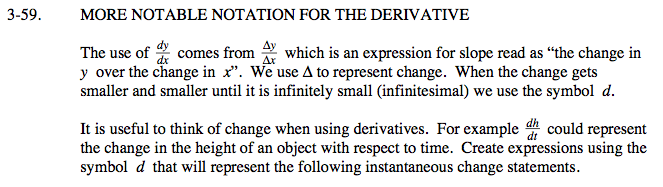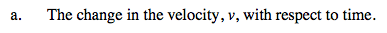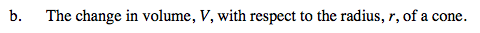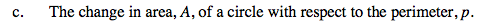### Home > CALC > Chapter 3 > Lesson 3.2.2 > Problem3-59

3-59.
1. MORE NOTABLE NOTATION FOR THE DERIVATIVE
The use of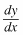comes from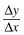which is an expression for slope read as "the change in y over the change in x". We use Δ to represent change. When the change gets smaller and smaller until it is infinitely small (infinitesimal) we use the symbol d.

It is useful to think of change when using derivatives. For example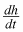could represent the change in the height of an object with respect to time. Create expressions using the symbol d that will represent the following instantaneous change statements. Homework Help ✎

1. The change in the velocity, v, with respect to time.

2. The change in volume, V, with respect to the radius, r, of a cone.

3. The change in area, A, of a circle with respect to the perimeter, p.